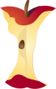Aligned To Common Core Standard:

Grade 3 Base Ten - 3.NBT.2

Printable Worksheets And Lessons

• Mixed Step-by-step Lesson- Start with an expanded form problem, then move on to a sum and a difference.

• Guided Lesson - These are pretty random. I was just trying to cover everything in a sort of shotgun approach, I guess.

• Guided Lesson Explanation - This one is a complete walk through for each problem.

• Practice Worksheet - We add and subtract vertically and then we ask kids to setup the problems themselves based on input / output chart.

• Matching Worksheet - Find the missing part of each problem.(Click Here to Upgrade)

Homework Sheets

One homework on each skill and the third sheet mixes them together.

Practice Worksheets

A direction based sheet, some matching, and some honest problems for you.

 Practice 1 Practice 2 Practice 3 Practice 4

Math Skill Quizzes

15 of each type of problem in a standard format.